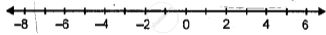# Fill in the Blanks, Using the Following Number Line: 8 is _______ than -5 and -8 is ______Than -5. - Mathematics

Fill in the Blanks

Fill in the blanks, using the following number line:8 is _______ than -5 and -8 is ______than -5.

#### Solution

8 is greater than -5 and -8 is less than -5.

Concept: Operation on of Whole Number on Number Line
Is there an error in this question or solution?

#### APPEARS IN

Selina Class 6 Mathematics
Chapter 7 Number Line
Exercise 7 (A) | Q 1.8